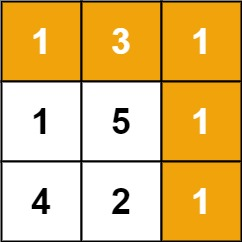# 64. Minimum Path Sum

Medium
Given a `m x n` `grid` filled with non-negative numbers, find a path from top left to bottom right, which minimizes the sum of all numbers along its path.
Note: You can only move either down or right at any point in time.
Example 1:Input: grid = [[1,3,1],[1,5,1],[4,2,1]]
Output:
7
Explanation:
Because the path 1 → 3 → 1 → 1 → 1 minimizes the sum.
Example 2:
Input: grid = [[1,2,3],[4,5,6]]
Output:
12
Constraints:
• `m == grid.length`
• `n == grid[i].length`
• `1 <= m, n <= 200`
• `0 <= grid[i][j] <= 100`

### 解題

func minPathSum(grid [][]int) int {
m, n := len(grid), len(grid)
dp := grid
for i := 1; i < m; i++ {
dp[i] = dp[i - 1] + grid[i]
}
for i := 1; i < n; i++ {
dp[i] = dp[i - 1] + grid[i]
}
for i := 1; i < m; i++ {
for j := 1; j < n; j++ {
dp[i][j] = min(dp[i - 1][j], dp[i][j - 1]) + grid[i][j]
}
}
return dp[m - 1][n - 1]
}
func min(a, b int) int {
if a < b {
return a
}
return b
}